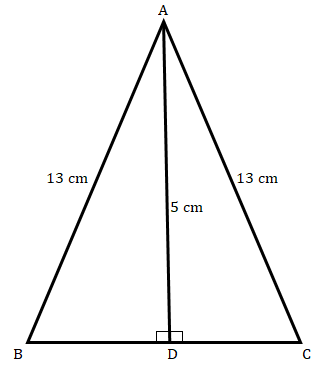# In an isosceles triangle $ABC$, if $AB\ =\ AC\ =\ 13\ cm$ and the altitude from $A$ on $BC$ is $5\ cm$, find $BC$."

Given:

In the given isosceles triangle $ABC$, $AB\ =\ AC\ =\ 13\ cm$ and the altitude from $A$ on $BC$ is $5\ cm$.

To do:

We have to find $BC$.

Solution:

In $∆ADB$,

By using Pythagoras theorem,

$AD^2 + BD^2 = AB^2$

$5^2 + BD^2 = 13^2$

$BD^2 = 169 – 25$

$BD^2= 144$

$BD = \sqrt{144} = 12\ cm$

Similarly,

In $∆ADC$,

By applying Pythagoras theorem,

$AC^2 = AD^2 + DC^2$

$13^2 = 5^2 + DC^2$

$DC^2 = 169-25$

$DC = \sqrt{144} = 12\ cm$

Therefore,

$BC = BD + DC = (12+12)\ cm = 24\ cm$

The length of $BC$ is $24\ cm$.